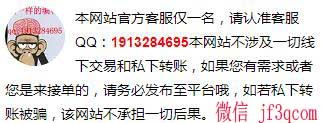# 链表有关问题，包括合并、删除，求大神帮忙

*若价格不公道，可以让提问者在平台追加赏金哦，平台是您利益的保证

Problem 2: transform(lst)
Given a linked list, transform it into a Python list. The head of the linked list corresponds to the left of the Python list. When the input linked list is empty, the output Python list should be empty as well.
Example: When the input linked list is 1 → 2 → 3, the output Python list should be [1,2,3] .

Problem 3: concatenate(lst1, lst2 )
Given two (possibly empty) linked lists, concatenate them such that the first list comes first.
Example: When the first input linked list lst1 is 1 → 2 → 3 and the second lst2 is 7 → 8 → 9, the output
linked list is 1 → 2 → 3 → 7 → 8 → 9.

Problem 4: removeNodesFromBeginning(lst, n)
Remove the first n nodes from a (possible empty) linked list.
Note: 0 ≤ n ≤ lst.length()
Example: Given an linked list 1 → 2 → 3 → 4 → 5 and n = 3, return the linked list 4 → 5.

Problem 5: removeNodes(lst, i, n)
Starting from the ith node in a (possibly empty) linked list, remove the next n nodes, not including the ith node.
• We say the head of the input list is the first node, i.e., i = 1.
• When i = 0, your function should start by removing the head. Note:
•n≥0
•i≥0
• i + n ≤ lst.length()
Explanation: The second node (i = 2) is 2. Removing the next three nodes (n = 3) means removing nodes 3, 4, and 5. The remaining ones are 1, 2, 6.
Explanation: Since i = 0, we remove the first three nodes (n = 3) from the beginning of lst , which are 1, 2, 3. The remaining ones are 4, 5, 6.

*若价格不公道，可以让提问者在平台追加赏金哦，平台是您利益的保证。你觉得当前的价格如何呢，奉上您珍贵的一票吧`class Queue:    def __init__(self):        self.items = []    def isEmpty(self):        return self.items == []    def enqueue(self, item):        self.items.insert(0, item)    def dequeue(self):        return self.items.pop()    def size(self):        return len(self.items)"""Node class used for Problem 2-5"""class Node:    def __init__(self, data):        self.data = data        self.next = None    def getData(self):        return self.data    def getNext(self):        return self.next    def setData(self, data):        self.data = data    def setNext(self, node):        self.next = node"""1. Stack2 classImplement stack data structure using queue"""class Stack2:    def __init__(self):        # Write your definition for __init__ here        self.items = []    def isEmpty(self):        # Write your definition for isEmpty here        return self.items == []    def push(self, item):        # Write your definition for push here        self.items.append(item)    def pop(self):        # Write your definition for pop here        if self.items:            return self.items.pop(0)        else:            print("pop() error: Stack is Empty.")            return None    def peek(self):        # Write your definition for peek here        if self.items:            return self.items[-1]        else:            print("pop() error: Stack is Empty.")            return None    def size(self):        # Write your definition for size here        return len(self.items)        """2. transform(lst)Transform an unordered list into a Python listInput: an (possibly empty) unordered listOutput: a Python list"""def transform(lst):    # Write your code here    res = []    if lst is None:        return res    while 1:        res.append(lst.getData())        if lst.getNext() is None: break        lst = lst.getNext()    return res"""3. concatenate(lst1, lst2)Concatenate two unordered listsInput: two (possibly empty) unordered listOutput: an unordered list"""def concatenate(lst1, lst2):    # Write your code here    if lst1 is None and lst2 is None:        return None    elif lst1 is None:        return lst2    elif lst2 is None:        return lst1    res = lst1    while 1:        if lst1.getNext() is None: break        lst1 = lst1.getNext()    lst1.setNext(lst2)        return res            # lst1 = [Node(1),Node(2),Node(3)]# lst1.setNext(lst1)# lst1.setNext(lst1)# lst2 = [Node(7),Node(8),Node(9)]# lst2.setNext(lst2)# lst2.setNext(lst2)# print(concatenate(lst1, lst2))"""4. removeNodesFromBeginning(lst, n)Remove the first n nodes from an unordered listInput:    lst -- an (possibly empty) unordered list    n -- a non-negative integerOutput: an unordred list"""def removeNodesFromBeginning(lst, n):    # Write your code here    if lst is None or n < 0:        return None        while n > 0:        if lst.getNext() is None: break        temp = lst        lst = lst.getNext()           temp.setNext(None)        n -= 1    if n == 0: return lst        # lst = [Node(1),Node(2),Node(3),Node(4),Node(5)]# lst.setNext(lst)# lst.setNext(lst)# lst.setNext(lst)# lst.setNext(lst)# print(removeNodesFromBeginning(lst, 3))"""5. removeNodes(lst, i, n)Starting from the ith node, remove the next n nodes(not including the ith node itself).Assume i + n <= lst.length(), i >= 0, n >= 0.Input:    lst -- an unrdered list    i -- a non-negative integer    n -- a non-negative integerOutput: an unordred listlst = [1, 2, 3, 4, 5]i = 2n = 2return [1, 2, 5]i = 1n = 2return [1, 4, 5]i = 0n = 2return [3, 4, 5]"""def removeNodes(lst, i, n):    # Write your code here    if lst is None or n < 0 or i < 0:        return None    res = lst    left = None    right = None    idx = 0    while 1:        if i > 0 and idx == i - 1:            left = lst        if idx == i + n:            right = lst            break        if lst.getNext() is None: break        lst = lst.getNext()           idx += 1        left and left.setNext(right)        if right and left is None : return right    elif left: return res# lst = [Node(1),Node(2),Node(3),Node(4),Node(5)]# lst.setNext(lst)# lst.setNext(lst)# lst.setNext(lst)# lst.setNext(lst)# removeNodes(lst,4, 1)`2021-01-27 16:03:00

##### 公告##### 第一时间了解动态##### 关注我们